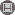Volume 12, Issue 4 (Vol.12, No.4 2017)                   irje 2017, 12(4): 55-63 | Back to browse issues page

BibTeX | RIS | EndNote | Medlars | ProCite | Reference Manager | RefWorks
Send citation to:Hamzeh S, Soltanian A, Faradmal J. Confidence Interval Estimation of Proportion Near Zero or One: A Modeling Secondary Study. irje. 2017; 12 (4) :55-63
URL: http://irje.tums.ac.ir/article-1-5621-en.html
1- کارشناسی ارشد گروه آمار زیستی و اپیدمیولوژی، دانشکده بهداشت، دانشگاه علوم پزشکی همدان، همدان، ایران
2- دانشیار آمار زیستی، گروه آمار زیستی، مرکز تحقیقات مدل‌سازی بیماری‌های غیر واگیر، دانشکده بهداشت، دانشگاه علوم پزشکی همدان، همدان، ایران , soltanian@umsha.ac.ir
3- دانشیار آمار زیستی، گروه آمار زیستی، مرکز تحقیقات مدل‌سازی بیماری‌های غیر واگیر، دانشکده بهداشت، دانشگاه علوم پزشکی همدان، همدان، ایران
Abstract:   (5263 Views)

Background and Objectives: When computing a confidence interval for a binomial proportion p, one must choose an exact interval that has a coverage probability of at least 1-α for all values of p. In this study, we compared the confidence intervals of Clopper-Pearson, Wald, Wilson, and double ArcSin transformation in terms of maintaining a constant nominal type I error.

Methods: Simulations were used to compare four methods of estimating a confidence interval, including the Clopper-Pearson, Wald, Wilson, and double ArcSic. The data were generated from the binomial and Poison distribution with parameters p, n and µ=np, 1000 were produced . Type I error of each method was calculated per simulation. The above methods were used to estimate confidence intervals in a meta-analysis study.

Results: The results of the simulation study showed that double ArcSin keep confidence interval at [0,1], but for some proportion has high type I error or low coverage probability. The Clopper–Pearson interval guarantees that the coverage probability is always equal to or above the nominal confidence level for any fixed p.

Conclusion: This study showed that confidence interval estimations the Clopper-Pearson than other methods of calculating the type I error fixed and smaller.